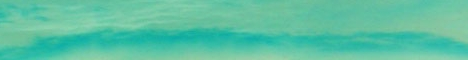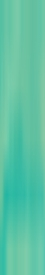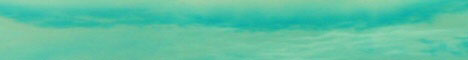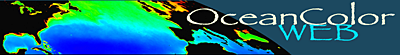Home NPP Products LIDAR Frequently Asked Questions Site Map Land / Ocean Merge Field / Lab Data Highlights / Results### what projection are these data in?

These hdf files are in an Equidistant Cylindrical projection.

### how do we convert rows and columns to lats and lons?

The rows represent lines of latitude, and the columns coincide with longitude.

For 1080 by 2160 data, the grid spacing is 1/6 of a degree in both latitude and longitude.

• 1080 rows * 1/6 degree per row = 180 degrees of latitude (+90 to -90).
• 2160 columns * 1/6 degree per column = 360 degrees of longitude (-180 to +180).

• The north west corner of the start of the hdf file is at +90 lat, -180 lon.

To obtain the location of the center of any pixel:
• take the number of rows and columns you are away from the NW corner,
• multiply by the grid spacing to get the change in latitude and longitude,
• subtract the change in latitude from +90 lat,
• add the change in longitude to -180 lon;
• shift the latitude down (subtract) by 1/2 of a grid spacing
• and shift the longitude over (add) by 1/2 of a grid spacing

• This gives the center of any pixel. To get the NW corner of any pixel, do not shift over and down by 1/2 of a grid spacing.

### how do we fill in gaps?

We use a gap-fill routine that was developed here. We search an expanding zone centered on the missing data until we find "good" values, and then average all available data in that zone to represent the missing value.
What makes this a bit more complex is that the same pixel 8days before and after will give a better estimate of the missing value than does looking further and further away from the pixel.

### do we gap-fill npp?

Although it seems natural to do so, it is actually incorrect to do it that way. If we did, we would be projecting "clear sky" npp values into cloud covered areas (ie, lower par levels), and the estimates would be off. Instead, we cloud fill all input data to the NPP algorithms, and then calculate the npp values.

### how do we get daylength?

daylength is a function of latitude and day of year.
Given those two variables, there are different algorithms available for this calculation.
If you do not have a preferred algorithm, the following is the function used here (in C):

 ``` float LatToDayLength(float lat, int yDay) { // get lat in radians double gamma = lat / 180.0 * M_PI; // convert date into an angle double psi = yDay / 365.0 * 2.0 * M_PI; // calc solar declination // Kirk page 35 double solarDec = (0.39637 - 22.9133 * cos(psi) + 4.02543 * sin(psi) - 0.38720 * cos(2*psi) + 0.05200 * sin(2*psi)) *M_PI / 180.0; double r = -tan(gamma) * tan(solarDec); if(r <= -1) return 24.0; else if(fabs(r) < 1) return 24.0 * acos(r) / M_PI; else return 0; } ```

### how do we calculate total production of carbon from a given npp file?

The units of the npp files are: mg C / m**2 / day

If everything were simply in mg C, then all you would have to do is sum up the pixels.

You will need to multiply each pixel by the number of days represented by the hdf file (either 8day or monthly).

You will also need to multiply each pixel by its area in square meters.
One degree on a great circle is about 111.1 km;
one pixel (for 1080x2160 files) is 1/6 of a degree.
At the equator, one pixel has an area of about (111.1 km / 6)*(111.1 km / 6),
and you need to scale this according to the latitude you are at:
pixel area = cos(latitude) * pixel area at equator
be sure to remember to convert from area in km**2 to area in m**2.

So, read in each pixel, multiply the pixel value by the area of the pixel in square meters and by the number of days the file represents, and sum over all pixels.